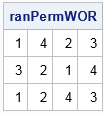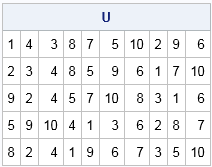A colleague and I recently discussed how to generate random permutations without encountering duplicates. Given a set of n items, there are n! permutations My colleague wants to generate k unique permutations at random from among the total of n!. Said differently, he wants to sample without replacement from the set of all possible permutations.

In SAS, you can use the RANPERM function to generate ranom permutations. For example, the statement p = ranperm(4) generates a random permutation of the elements in the set {1, 2, 3, 4}. However, the function does not ensure that the permutations it generates will be unique.

I can think of two ways to generate random permutations without replacement. The first is to generate all permutations and then choose a random subset of size k without replacement from the set of n! possibilities. This technique is efficient when n is small or when k is a substantial proportion of n!. The second technique is to randomly generate permutations until you get a total of k that are unique. This technique is efficient when n is large and k is small relative to n!.

### Generate all permutations, then sample without replacement

For small sets (say, n ≤ 8), an efficient way to generate random permutations is to generate all permutations and then extract a random sample without replacement. In the SAS/IML language you can use the ALLPERM function to generate all permutations of a set of n items, where n ≤ 18. The ALLPERM function returns a matrix that has n! rows and n columns. Each row is a permutation. You can then use the SAMPLE function to sample without replacement from among the rows, as follows:

```proc iml; call randseed(123); n = 4; /* permutations of n items */ k = 3; /* want k unique permutation */   p = allperm(n); /* matrix has n! rows; each row is a permutation */ rows = sample(1:n, k, "WOR"); /* random rows w/o replacement */ ranPermWOR = p[rows, ]; /* extract rows */ print ranPermWOR;```### Generate random permutations, then check for uniqueness

The matrix of all permutations has n! rows, which gets big fast. If you want only a small number of permutations from among a huge set of possible permutations, it is more efficient to use the RANPERM function to generate permutations, then discard duplicates. Last week I showed how to eliminate duplicate rows from a numeric matrix so that the remaining rows are unique. The following SAS/IML statements use the UniqueRows function, which is defined in the previous post. You must define or load the module before you can use it.

```/* <define or load the DupRows and UniqueRows modules HERE> */   n = 10; /* permutations of n items */ k = 5; /* want k unique permutation; k << n! */ p = ranperm(n, 2*k); /* 2x more permutations than necessary */ U = UniqueRows(p); /* get unique rows of 2k x n matrix */ if nrow(U) >= k then U = U[1:k, ]; /* extract the first k rows */ else do; U = UniqueRows( U // ranperm(n, 10*k) ); /* generate more... repeat as necessary */ if nrow(U) >= k then U = U[1:k, ]; /* extract the first k rows */ end; print U;```Notice in the previous statements that the call to RANPERM requests 2*k random permutations, even though we only want k. You should ask for more permutations than you need because some of them might be duplicates. The factor of 2 is ad hoc; it is not based on any statistical consideration.

If k is much smaller than n!, then you might think that the probability of generating duplicate a permutation is small. However, the Birthday Problem teaches us that duplicates arise much more frequently than we might expect, so it is best to expect some duplicates and generate more permutations than necessary. When k is moderately large relative to n!, you might want to use a factor of 5, 10, or even 100. I have not tried to compute the number of permutations that would generate k unique permutations with 95% confidence, but that would be a fun exercise. In my program, if the initial attempt generates fewer than k unique rows, it generates an additional 10*k permutations. You could repeat this process, if necessary.

In summary, if you want to generate random permutations without any duplicates, you have at least two choices. You can generate all permutations and then extract a random sample of k rows. This method works well for small values of n, such as n ≤ 8. For larger values of n, you might want to generate random permutation (more than k) and use the first k unique rows of the matrix of permutations.

The post Random permutations without duplicates appeared first on The DO Loop.